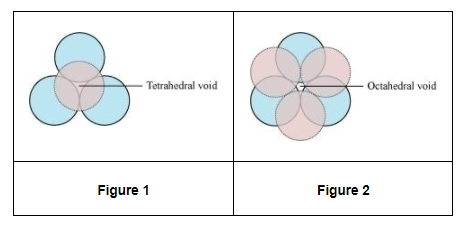# How will you distinguish between the following pairs of terms:

Question:

How will you distinguish between the following pairs of terms:

(i) Hexagonal close-packing and cubic close-packing?

(ii) Crystal lattice and unit cell?

(iii) Tetrahedral void and octahedral void?

Solution:

i. A 2-D hexagonal close-packing contains two types of triangular voids (a and b) as shown in figure 1. Let us call this 2-D structure as layer A. Now, particles are kept in the voids present in layer A (it can be easily observed from figures 2 and 3 that only one of the voids will be occupied in the process, i.e., either a or b). Let us call the particles or spheres present in the voids of layer A as layer B. Now, two types of voids are present in layer B (c and d). Unlike the voids present in layer A, the two types of voids present in layer B are not similar. Void c is surrounded by 4 spheres and is called the tetrahedral void. Void d is surrounded by 6 spheres and is called the octahedral void.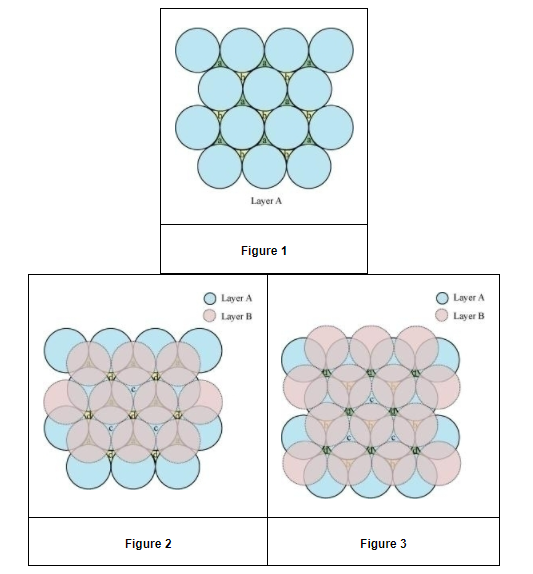Now, the next layer can be placed over layer B in 2 ways.

Case 1: When the third layer (layer C) is placed over the second one (layer B) in such a manner that the spheres of layer C occupy the tetrahedral voids c.

In this case we get hexagonal close-packing. This is shown in figure 4. In figure 4.1, layer B is present over the voids a and layer C is present over the voids c. In figure 4.2, layer B is present over the voids b and layer C is present over the voids c. It can be observed from the figure that in this arrangement, the spheres present in layer C are present directly above the spheres of layer A. Hence, we can say that the layers in hexagonal close-packing are arranged in an ABAB….. pattern.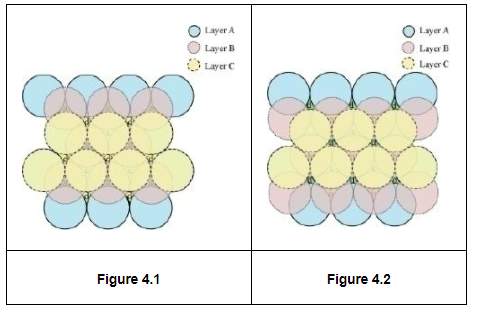Case 2: When the third layer (layer C) is placed over layer B in such a manner that the spheres of layer C occupy the octahedral voids d.

In this case we get cubic close-packing. In figure 5.1, layer B is present over the voids a and layer C is present over the voids d. In figure 5.2, layer B is present over the voids b and layer C is present over the voids d. It can be observed from the figure that the arrangement of particles in layer C is completely different from that in layers A or B. When the fourth layer is kept over the third layer, the arrangement of particles in this layer is similar to that in layer A. Hence, we can say that the layers in cubic close-packing are arranged in an ABCABC….. pattern.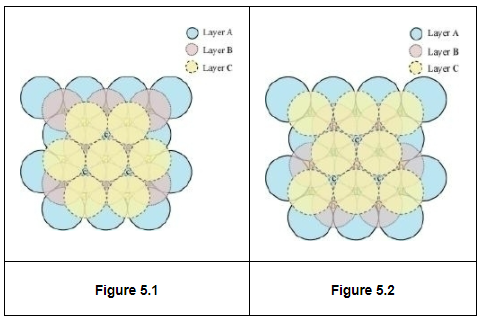The side views of hcp and ccp are given in figures 6.1 and 6.2 respectively.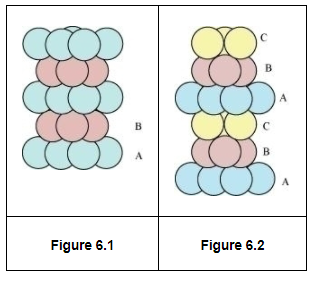(ii) The diagrammatic representation of the constituent particles (atoms, ions, or molecules) present in a crystal in a regular three-dimensional arrangement is called crystal lattice.

A unit cell is the smallest three-dimensional portion of a crystal lattice. When repeated again and again in different directions, it generates the entire crystal lattice.

(iii) A void surrounded by 4 spheres is called a tetrahedral void and a void surrounded by 6 spheres is called an octahedral void. Figure 1 represents a tetrahedral void and figure 2 represents an octahedral void.